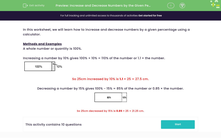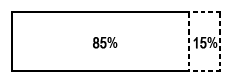# Increase and Decrease Numbers by a Given Percentage

In this worksheet, students will practise increasing and decreasing numbers by the given percentages. They can use a calculator to help.Key stage:  KS 3

Curriculum topic:   Number

Curriculum subtopic:   Define, Interpret and Compare Percentages

Difficulty level:#### Worksheet Overview

In this activity, we will learn how to increase and decrease numbers by a given percentage using a calculator.

Methods and Examples

A whole number or quantity is 100%.

Increasing a number by 10% gives 100% + 10% = 110% of the number or 1.1 × the number.So 25 cm increased by 10% is 1.1 × 25 = 27.5 cm.

Decreasing a number by 15% gives 100% - 15% = 85% of the number or 0.85 × the number.So 25 cm decreased by 15% is 0.85 × 25 = 21.25 cm.

Let's try one more example of each, shall we?

What is 73% increased by 25%? 25% extra means 100% plus 25% which makes 125%. So we need to multiply by 1.25, we say that our multiplier is 1.25.

73 x 1.25 = 91.25

What if 73% decreased by 16%?

100 - 16 = 84, so our multiplier becomes 0.84

73 x 0.84 = 61.32

Does this make sense? Let's have a go at some questions then and if you need to check back to these instructions, just click on the red button at any point.### What is EdPlace?

We're your National Curriculum aligned online education content provider helping each child succeed in English, maths and science from year 1 to GCSE. With an EdPlace account you’ll be able to track and measure progress, helping each child achieve their best. We build confidence and attainment by personalising each child’s learning at a level that suits them.

Get started